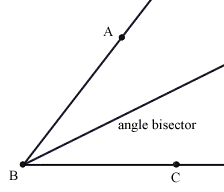index: click on a letter A B C D E F G H I J K L M N O P Q R S T U V W X Y Z A to Z index index: subject areas numbers & symbols sets, logic, proofs geometry algebra trigonometry advanced algebra & pre-calculus calculus advanced topics probability & statistics real world applications multimedia entrieswww.mathwords.com about mathwords website feedback

 Angle Bisector A line or ray that divides an angle in half. For polygons, an angle bisector is a line that bisects an interior angle. Note: The angle bisectors of a triangle are concurrent and intersect at the incenter.Angle bisector of ∠ABC (Click on the image or here to launch an interactive java applet)   See also Incircle, centers of a triangle